# Pricing bonds with different cash flows and compounding frequencies

Explore how bond rates and payments are formulated.

•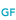WILEY GLOBAL FINANCE

Equation 1 defines the value of a bond that pays coupons on an annual basis and a principal at maturity.

The value of a bond paying a fixed coupon interest each year (annual coupon payment) and the principal at maturity, in turn, would be:

Equation 1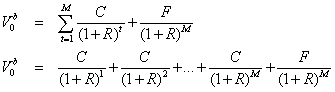Where M = Number of years to maturity

With the coupon payment fixed each period, the C term in Equation 1 can be factored out and the bond value can be expressed as: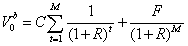Bonds, of course, differ in the frequency in which they pay coupons each year, and many bonds have maturities less than one year. Also, when investors buy bonds they often do so at non-coupon dates. Equation 1, therefore, needs to be adjusted to take these practical factors into account.

## Semiannual coupon payments

Many bonds pay coupon interest semiannually. When bonds make semiannual payments, 3 adjustments to Equation 1 are necessary: (1) the number of periods is doubled; (2) the annual coupon rate is halved; (3) the annual discount rate is halved. Thus, if our illustrative 10-year, 9% coupon bond trading at a quoted annual rate of 10% paid interest semiannually instead of annually, it would be worth \$937.69: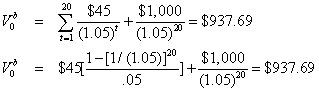Note that the rule for valuing semiannual bonds is easily extended to valuing bonds paying interest even more frequently. For example, to determine the value of a bond paying interest 4 times a year, we would quadruple the periods and quarter the annual coupon payment and discount rate. In general, if we let n be equal to the number of payments per year (i.e., the compoundings per year), M be equal to the maturity in years, RA be the discount rate quoted on an annual basis (simple annual rate), and R be equal to the periodic rate, then we can express the general formula for valuing a bond as follows: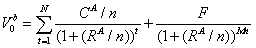CA = annual coupon = (CR)(F)
n = number of payments per year
Periodic coupon = Annual coupon/n
M = term to maturity in years
N = number of periods to maturity = (n)(M)
Required periodic rate = R = Annual rate/n =
RA/n

Thus, the value of a 20-year, 6% coupon bond, with semiannual payments, a par value of \$1,000, and a required return of 8% would be \$802.07: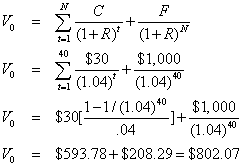N = number of periods = 40 [= (20 years)(2)]
F = \$1,000
C = semiannual coupon = (.06/2)(\$1,000) = \$30
R = required semiannual rate = .08/2 = .04

## Compounding frequency

The 10% annual rate in the first example and the 8% rate in the second is a simple annual rate: It is the rate with one annualized compounding. With one annualized compounding and a 10% annual rate, we earn 10% every year and a \$100 investment would grow to equal \$110 after one year: \$100(1.10) = \$110. If the simple annual rate were expressed with semiannual compounding, then we would earn 5% every 6 months with the interest being reinvested; in this case, \$100 would grow to equal \$110.25 after one year: \$100(1.05)2 = \$110.25. If the rate were expressed with monthly compounding, then we would earn 0.8333% (10%/12) every month with the interest being reinvested; in this case, \$100 would grow to equal \$110.47 after one year: \$100[1 + (.10/12)]12 = \$110.47. If we extend the compounding frequency to daily, then we would earn 0.0274% (10%/365) daily, and with the reinvestment of interest, a \$100 investment would grow to equal \$110.52 after one year: \$100[1 + (.10/365)]365 = \$110.52. Note that the rate of 10% is the simple annual rate, whereas the actual rate earned for the year is [1+(RA/n)]n-1. This rate that includes the reinvestment of interest (or compounding) is known as the effective rate.

When the compounding becomes large, such as daily compounding, then we are approaching continuous compounding with the n term in the above equation becoming very large. For cases in which there is continuous compounding, the future value (FV) for an investment of A dollars M years from now is equal to: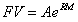where e is the natural exponent (equal to the irrational number 2.71828). Thus, if the 10% simple rate were expressed with continuous compounding, then \$100 (A) would grow to equal \$110.52 after one year: \$100e(.10)(1) = \$110.52. (After allowing for some slight rounding differences, this is the value obtained with daily compoundings.) After 2 years, the \$100 investment would be worth \$122.14: \$100e(.10)(2) = \$122.14.

Note that from the FV expression, the present value (A) of a future receipt (FV) is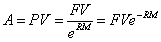If R = .10, a security paying \$100 2 years from now would be worth \$81.87, given continuous compounding: PV = \$100e(.10)(2) = \$81.87. Similarly, a security paying \$100 each year for 2 years would be currently worth \$172.36: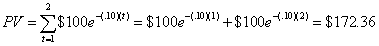Thus, if we assume continuous compounding and a discount rate of 10%, then the value of our 10-year, 9% bond would be \$908.82: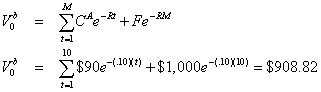It should be noted that most practitioners use interest rates with annual or semiannual compounding. Most of our examples, in turn, will follow that convention. However, continuous compounding is often used in mathematical derivations, and we will make some use of it when it is helpful.